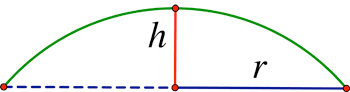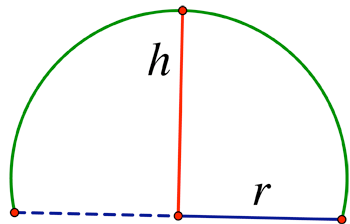SEARCH HOMEMath Central Quandaries & QueriesQuestion from sravya, a student: how to calculate surface area of a dome if its base radius and height are known and neither it is hemisphere nor radius of sphere is known??Hi Sravya,

Here is a method to get started.

Think of the height and the diameter (double the radius) as giving 3 points in the plane. There is a unique circle for three such points in the plane.or perhaps:That circle, rotated, gives the equation of the dome. So now you have the dome - you proceed to calculate the surface area.

(If you know calculus, you can also calculate the surface area of revolution from the cross-section circle).

Walter WhiteleyMath Central is supported by the University of Regina and The Pacific Institute for the Mathematical Sciences.